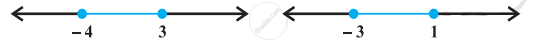# Solutions of the inequalities comprising a system in variable x are represented on number lines as given below, then ______. - Mathematics

MCQ
Fill in the Blanks

Solutions of the inequalities comprising a system in variable x are represented on number lines as given below, then ______.#### Options

• x ∈ (–oo, –4] ∪ [3, oo)

• x ∈ [–3, 1]

• x ∈ (–oo, –4) ∪ [3, oo)

• x ∈ [–4, 3]

#### Solution

Solutions of the inequalities comprising a system in variable x are represented on number lines as given below, then x ∈ (–∞, –4] ∪ [3, ∞).

Explanation:

x ∈ (–, –4] ∪ [3, ) is the correct choice. Common solution of the inequalities is from –to –4 and 3 to .

Concept: Algebraic Solutions of Linear Inequalities in One Variable and Their Graphical Representation
Is there an error in this question or solution?

#### APPEARS IN

NCERT Mathematics Exemplar Class 11
Chapter 6 Linear Inequalities
Solved Examples | Q 12 | Page 105
Share